Q.1. (a) Lenz’s law obeys the law of conservation of energy. Explain.
(b) Describe construction & working of a.c. generator.

Ans ⇒ (a) Lenz’s law : According to this law in the phenomenon of electromagnetic induction the direction of induced current is such that it opposes the cause by which it is produced. We see that in electromagnetic induction there is flow of current even in the abxence of cell or battery. Now question arises from where energy comes. There is transformation of other form of energy into electrical energy.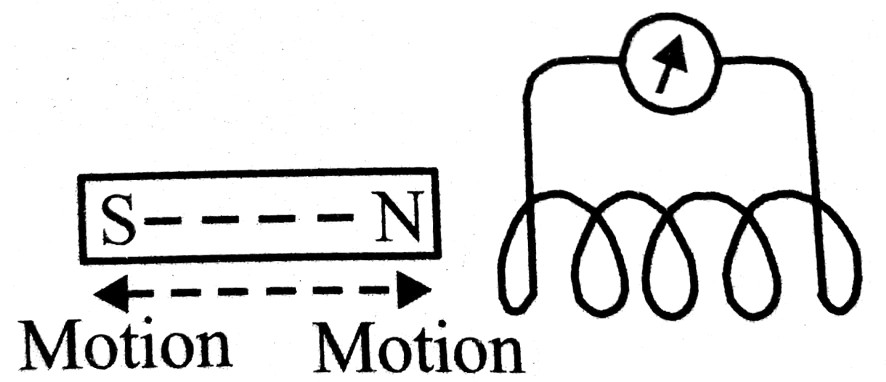By experiment it is found that the direction of current always opposes the moving magnet or primary coil. When magnet is brought near the coil, current produced in coil always opposes the motion of magnet. So work is to be done against this opposition. This workdone for opposition is stored in coil in form of electromagnetic potential energy.
On the other hand, when magnet is taken away the attraction is experienced because current is reversed. Again work is to be done. This work is transformed in electrical energy. hence we find that Lenz’s law is in accordance with law of conservation of energy.

(b) a.c. generator or dynamo : It is an electric instrument which converts mechanical energy into electrical energy. It is mainly based on the principle of electromagnetic induction.
The transformation of mechanical energy to electrical energy takes place in the form of a.c.

Construction : The apparatus has been shown in fig. It has following main parts :
(i) Field magnet : There are two pole piece of a strong magnet face to face. An uniform magnetic force field is produced between poles.

(ii) Armature : It is made by winding a copper coil (ABCD) on a cylindrical core of soft iron. It is fitted iwth insulated axle which is rotated between N & S by an instrument.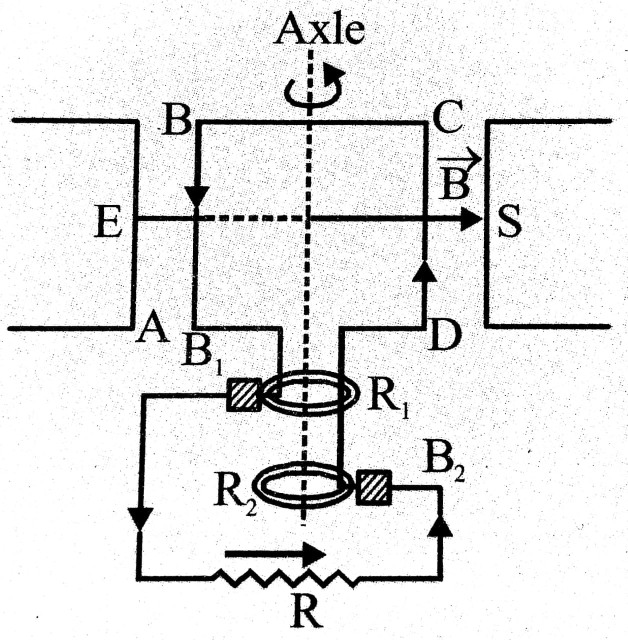(iii) Slipy rings : There are two rings R, & R, on axle to which two ends of ermature are joint separately. When axle rotates then rings move with armature.

(iv) Brush : Two brushes B, and B, of carbon touch the two rings R, & R..

Theory & working : When axle is rotated by a device, rectangular coil rotates in magnetic field. these is change in magnetic flux associated with it due to which an ac emfis generated in coil by electromagnetic induction. So, alternating current flow. If number of turns in coil in n, area of coil is A & magnetic field is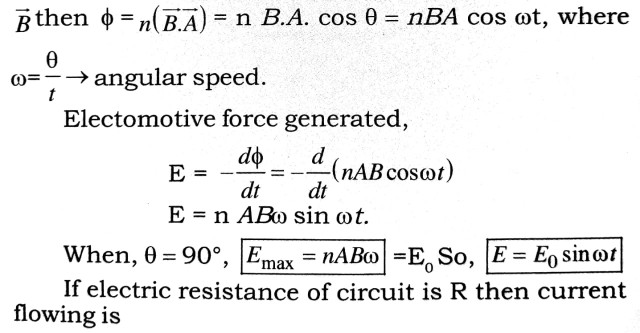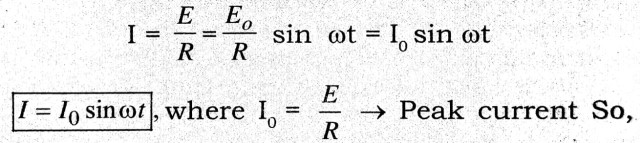current will be alternating.
If initially coil is I to the plane of paper & AB is up with CD down and coil is rotating in anticlockwise direction then in first half revolution current in AB will from B to A & in CD from D to C, (according to Fleming’s right hand screw rule).
After half angle AB & CD positions are interchanged. So current will be from C to Din CD & A to B in AB but in R it will be from B2 to B1.

Q.2. (a) What is electromagnetic induction ? State Faraday’s law of Electro magnetic induction.
(b) What do you mean by self induction & mutual induction ?

Ans ⇒ (a) Electromagnetic Induction : When magnetic flux linked with a loop charges, an emf is induced in the loop. This phenomenon is known as electromagnetic induction. In microscopic form, the production of induced electric field at a point due to the changing magnetic field at the same point, is the essence of electromagnetic induction.

Faraday’ Laws of EMI : The rate of decrement of magnetic flux is equal to the emf induced in a loop.
Let φ be the flux linked with the loop. Then –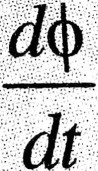= εind
Where, εind is the emf ‘induced’.
If Ē be the induced electric field intensity and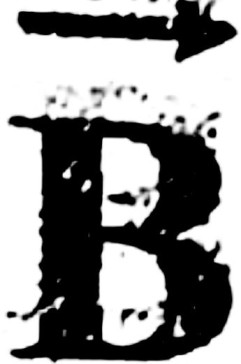be the magnetic induction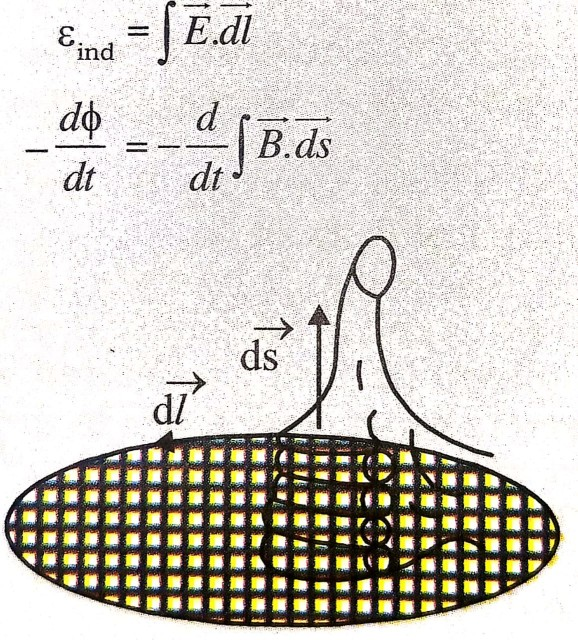(b) Self induction : When there is change in current flowing throught a closed coil, there is induction of an e.m.f. & electric current in circuit. These are called induced e.m.f. & induced current & the phenomenon is called self induction.

If no. of turns in coil are N & flux linked with each turn is φ, then total flux linked = Nφ

If current flowing through the coil is I then Nφ,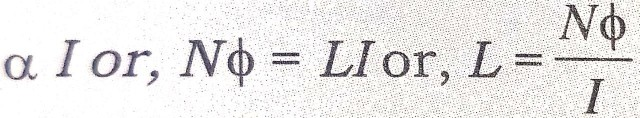Here, ∠is a constant called coefficient of self induction or self inductance.                              If I = 1 amp. then ∠Nφ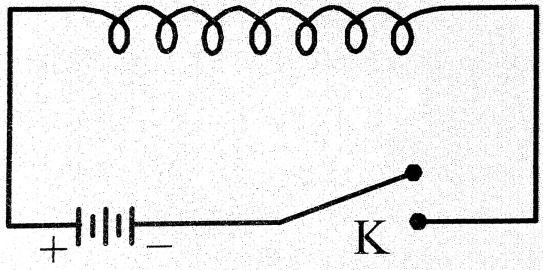i.e., self inductance is total flux produced by flow of unit current. Now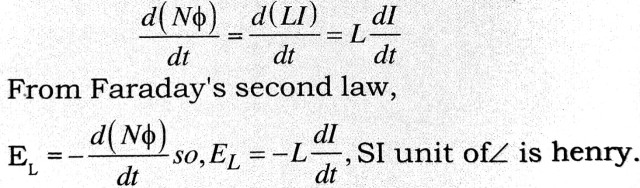Mutual induction : It is phenomenon of induction of an e.m.f. in second coil due to change in current through first coil situated near it.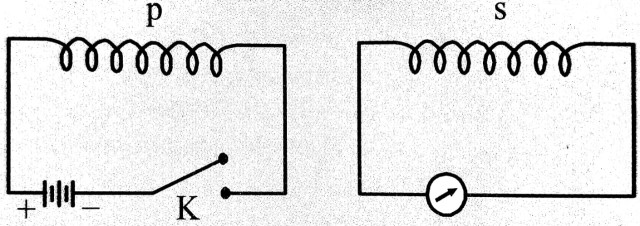The flux linked with secondary coil is directly proportional to flowing current.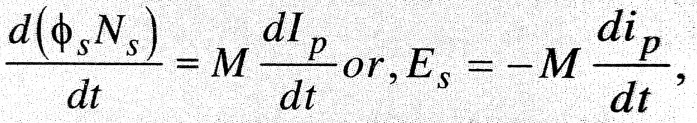Here M is a constant called ‘coefficient of mutual induction or mutual inductance. Its SI unit is henry.

Q.3. State Lenz’s law and show that it is in accordance of conservation law of energy.

Ans ⇒ Lenz’s Law : The direction of induced e.m.f. and induced current can be found from Lenz’s law whch can be stated as follows. The induced current and e.m.f. are in such a direction as to provent any change in the net number of lines of flux that pass throguh the corss-sectional area of the circuit Fig. That is, the induced current tends to maintain the original flux through the circuit. It can also be stated that the induced current has tendency to oppose the cause of production.
When a magnet having its north pole towards a loop is moved towards the loop, to oppose the motion of the magnet a north pole is developed which causes anti-clockwise current in the loop. If magnet is moved away from the loop, to oppose the motion of the
clockwise current in the loop. Similarly, when magnet having south pole towards the loop, approaches the loop, a south pole is developed which causes clockwise current in the loop and when the magnet is moved away, a north pole is developed to produce anticlockwise current in the loop as shown in figure.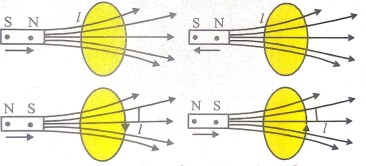Lenz’s law and law of conservation of energy : Electricity is a form of potential energy and work must be done to produce it when a N-pole approaches a coil, the direction o the induced current in the upper face gives rise to an induced N-pole. There is a repulsive force between the nearer ends of the magnet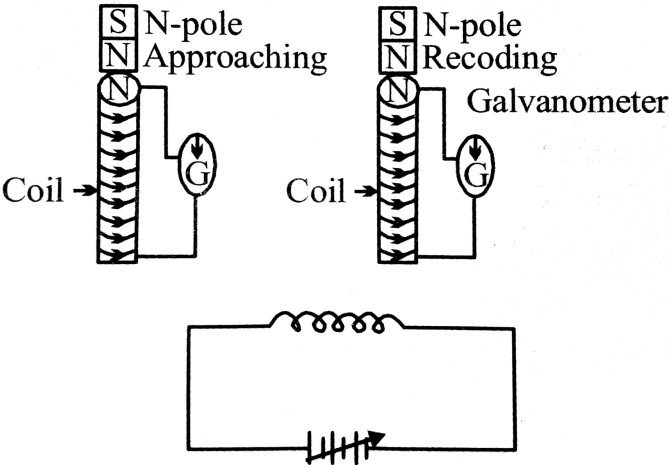and the coil. Work has to be done against this force of repulsion when the magnet approaches the coil. Similarly, when N-pole is withdrawn the pole developed at the new face is south pole, so that work has to be done against the force of attraction, to move the magnet. It is the mechanical energy spent by the outside system which is converted into the electrical energy stored in the coil. Thus we get electrical energy at the cost of mechanical energy spent. Hence Lenz’s law is in accordance with law of conservation of energy (fig.).
.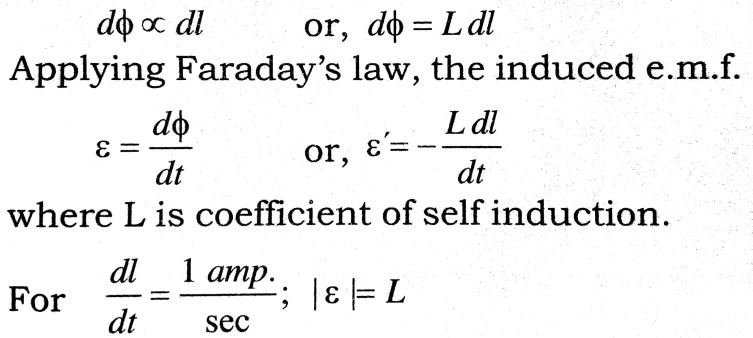∴       The coefficient of self induction is defined as the induced e.m.f. developed in a circuit in which current charges at the rate of one ampere per second.

Coefficient of self-induction for a solenoid : et change in current a solenoid of length l, number of turns N and area of cross-section A in time dt be dl (fig.) then the induced e.m.f. in the solenoid,
.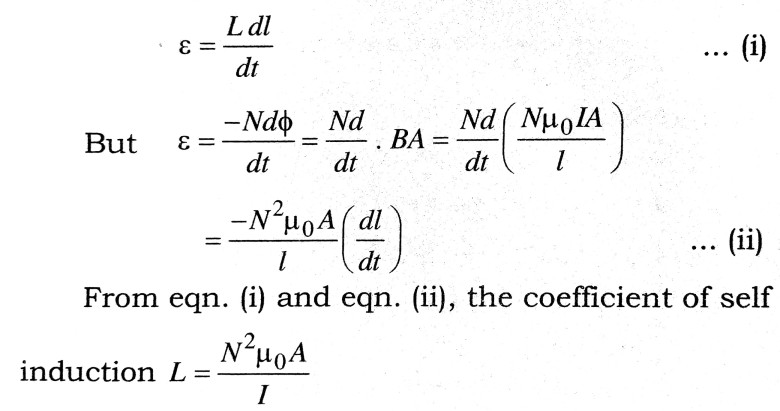Coefficient of self induction for a loop : Let dl be the change in current in a circular loop of radius and number turns N in time dt (fig.), then the induced e.m.f.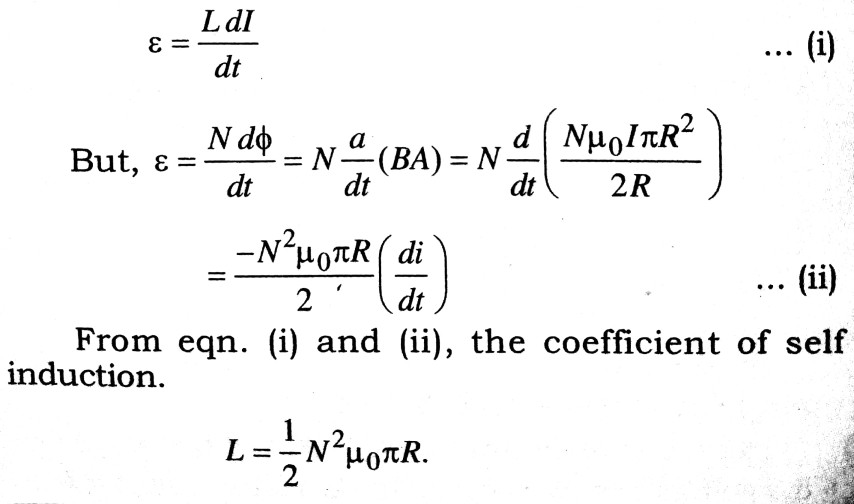## Class 12th physics Long Type question in English

 S.N Physics Long Type Question English Medium 1. ELECTRIC CHARGES AND FIELDS 2. LECTROSTATIC POTENTIAL AND CAPACITANCE 3. 4. 5. MAGNETISM AND MATTER 6. ELECTROMAGNETIC INDUCTION 7. ALTERNATING CURRENT 8. ELECTROMAGNETIC WAVES 9. RAY OPTICS AND OPTICAL INSTRUMENTS 10. WAVE OPTICS 11. DUAL NATURE OF MATTER AND RADIATION 12. ATOMS 13. NUCLEI 14. SEMI CONDUCTOR ELECTRONICS 15. COMMUNICATION SYSTEMS